Main Content

# cqt

Constant-Q nonstationary Gabor transform

## Syntax

``cfs = cqt(x)``
``[cfs,f] = cqt(x)``
``[cfs,f,g,fshifts] = cqt(x)``
``[cfs,f,g,fshifts,fintervals] = cqt(x)``
``[cfs,f,g,fshifts,fintervals,bw] = cqt(x)``
``[___] = cqt(___,Name,Value)``
``cqt(___)``

## Description

example

````cfs = cqt(x)` returns the constant-Q transform (CQT), `cfs`, of the input signal `x`. The input signal must have at least four samples. If `x` is a vector, then `cqt` returns a matrix corresponding to the CQT.If `x` is a matrix, then `cqt` obtains the CQT for each column (independent channel) of `x`. The function returns a multidimensional array corresponding to the maximally redundant version of the CQT. ```

example

````[cfs,f] = cqt(x)` returns the approximate bandpass center frequencies, `f`, corresponding to the rows of `cfs`. The frequencies are ordered from 0 to 1 and are in cycles/sample.```
````[cfs,f,g,fshifts] = cqt(x)` returns the Gabor frames, `g`, used in the analysis of `x` and the frequency shifts, `fshifts`, in discrete Fourier transform (DFT) bins between the passbands in the rows of `cfs`.`cfs`, `g`, and `fshifts` are required inputs for the inversion of the CQT with `icqt`.```
````[cfs,f,g,fshifts,fintervals] = cqt(x)` returns the frequency intervals, `fintervals`, corresponding the rows of `cfs`. The `k`th element of `fshifts` is the frequency shift in DFT bins between the `((k-1) mod N)` and` (k mod N)` element of `fintervals` with `k = 0,1,2,...,N-1` where `N` is the number of frequency shifts. Because MATLAB® indexes from 1, `fshifts(1)` contains the frequency shift between `fintervals{end}` and `fintervals{1}`, `fshifts(2)` contains the frequency shift between `fintervals{1}` and `fintervals{2}`, and so on.```
````[cfs,f,g,fshifts,fintervals,bw] = cqt(x)` returns the bandwidth, `bw`, in DFT bins of the frequency intervals, `fintervals`.```

example

````[___] = cqt(___,Name,Value)` returns the CQT with additional options specified by one or more `Name,Value` pair arguments, using any of the preceding syntaxes.```

example

````cqt(___)` with no output arguments plots the CQT in the current figure. Plotting is supported for vector inputs only. If the input signal is real and `Fs` is the sampling frequency, the CQT is plotted over the range `[0,Fs/2]`. If the signal is complex, the CQT is plotted over the range [`0,Fs`). NoteIn order to visualize a sparse CQT, coefficients have to be interpolated. When interpolation occurs, the plot can have significant smearing and be difficult to interpret. If you want to plot the CQT, we recommend using the default `TransformType` value `'full'`. ```

## Examples

collapse all

Load a signal and obtain the constant-Q transform.

```load noisdopp cfs = cqt(noisdopp);```

Load a real-valued signal and obtain the constant-Q transform. Return the approximate bandpass center frequencies.

```load handel [cfs,f] = cqt(y);```

Plot on a logarithmic scale the bandpass center frequencies through the Nyquist frequency.

```lfreq = length(f); nyquistBin = floor(lfreq/2)+1; plot(f(1:nyquistBin)) title('Bandpass Center Frequencies') grid on set(gca,'yscale','log')```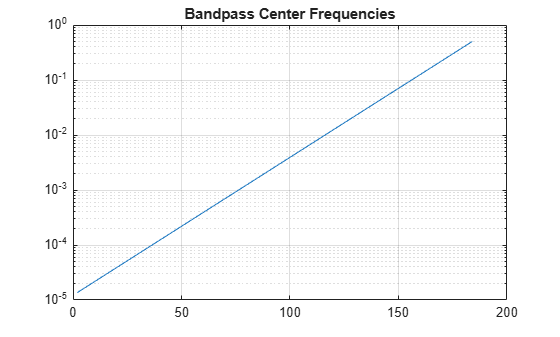To confirm the ratios of consecutive pairs of frequencies are constant, plot the ratios. Since `cqt` uses 12 bins per octave by default, the ratio should equal ${2}^{1/12}$. Since the DC and Nyquist frequencies are not members of the geometric sequence of center frequencies but are included in the frequency vector, exclude them from the plot.

```figure plot(f(3:nyquistBin-1)./f(2:nyquistBin-2)) grid on title(['Ratio: ',num2str(2^(1/12))])```Obtain the minimally redundant constant-Q transform of an audio signal. Use the Blackman-Harris window as the prototype function for the Gabor frames.

```load handel df = Fs/numel(y); [cfs,f,g,fshifts,fintervals,bw] = cqt(y,'SamplingFrequency',Fs,'TransformType',"sparse",'Window',"blackmanharris");```

`cfs` is a cell array, where each element in the array corresponds to a bandpass center frequency and Gabor frame. Plot the Gabor frame associated with the Nyquist frequency.

```lf = length(f); ind = floor(lf/2)+1; gFrame = fftshift(g{ind}); fvec = f(ind-1):df:f(ind+1)-df; plot(fvec,gFrame) xlabel('Frequency (Hz)') grid on title({['Gabor Frame - Freq: ',num2str(f(ind)),' Hz'];['Bandwidth ',num2str(bw(ind)*Fs/numel(y)),' Hz']})```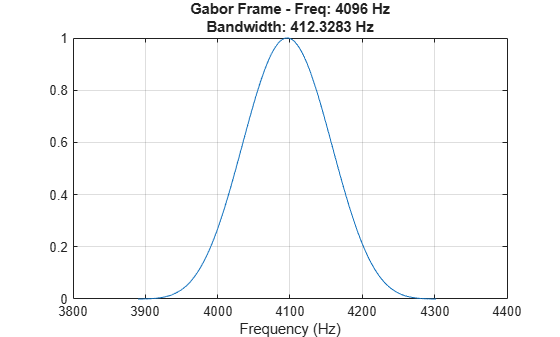In the constant-Q transform, the Gabor frames are applied to the discrete Fourier transform of the input signal, and the inverse discrete Fourier transform is performed. The k-th Gabor frame is applied to the k-th frequency interval specified in `fintervals`. Take the discrete Fourier transform of the signal and plot its magnitude spectrum. Use `fintervals` to indicate over which Fourier coefficients are the Gabor frame associated with the Nyquist frequency are applied.

```yDFT = fft(y); lyDFT = length(yDFT); plot(Fs*(0:lyDFT-1)/lyDFT,abs(yDFT)) grid on fIntervalGabor = fintervals{ind}; mx = max(abs(yDFT)); hold on plot([df*fIntervalGabor(1) df*fIntervalGabor(1)],[0 mx],'r-','LineWidth',2) plot([df*fIntervalGabor(end) df*fIntervalGabor(end)],[0 mx],'r-','LineWidth',2) str = sprintf('Gabor Frame Interval (Hz): [%3.2f, %3.2f]',df*fIntervalGabor(1),df*fIntervalGabor(end)); title(str)```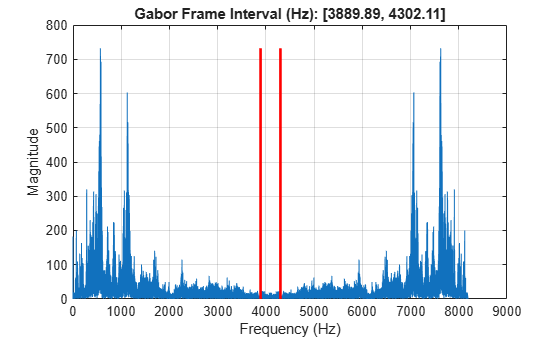Window the Fourier coefficients in the interval with the Gabor frame, and take the inverse discrete Fourier transform. Normalize the result, and compare with computed constant-Q coefficients and confirm they are equal.

```lGframe = length(gFrame); indx = 1:lGframe; indx = fftshift(indx); winDFT(indx) = yDFT(fIntervalGabor).*fftshift(gFrame(indx)); cqCoefs = ifft(winDFT); cqCoefs = (2*lGframe/length(y))*cqCoefs; max(abs(cqCoefs(:)-cfs{ind}(:)))```
```ans = 0 ```

Load an audio signal. Plot the constant-Q transform (CQT) using the maximally redundant version of the transform and using 12 bins per octave.

```load handel cqt(y,'SamplingFrequency',Fs)```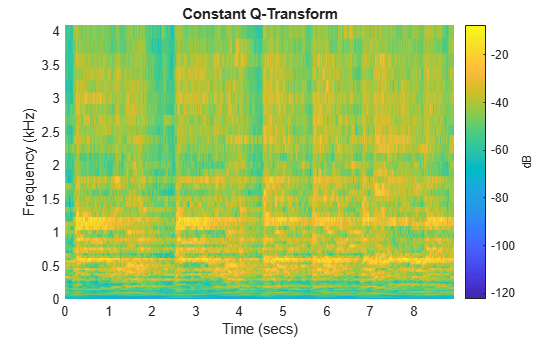Perform the CQT of the same signal using 48 bins per octave. Set the frequency range over which the CQT has a logarithmic frequency response to be the minimum allowable frequency to 2 kHz.

```minFreq = Fs/length(y); maxFreq = 2000; figure cqt(y,'SamplingFrequency',Fs,'BinsPerOctave',48,'FrequencyLimits',[minFreq maxFreq])```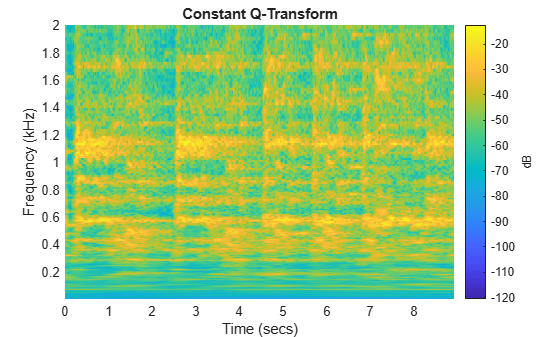## Input Arguments

collapse all

Input signal, specified as a real or complex vector or matrix. `x` must have at least four samples.

Data Types: `double`
Complex Number Support: Yes

### Name-Value Pair Arguments

Specify optional comma-separated pairs of `Name,Value` arguments. `Name` is the argument name and `Value` is the corresponding value. `Name` must appear inside quotes. You can specify several name and value pair arguments in any order as `Name1,Value1,...,NameN,ValueN`.

Example: `'SamplingFrequency',20,'BinsPerOctave',15`

Sampling frequency, in Hz, specified as the comma-separated pair consisting of `'SamplingFrequency'` and a positive scalar.

Number of bins per octave to use in the CQT, specified as a positive integer from 1 to 96.

Type of constant-Q transform to perform, specified as the comma-separated pair consisting of `'TransformType'` and `'full'` or `'sparse'`. The sparse transform is the minimally redundant version of the constant-Q transform.

Frequency limits over which the CQT has a logarithmic frequency response with the specified number of frequency bins per octave, specified as the comma-separated pair `'FrequencyLimits'` and a two-element real vector.

• The first element must be greater than or equal to `Fs/N`, where `Fs` is the sampling frequency and `N` is the length of the signal.

• The second element must be strictly less than the Nyquist frequency.

Window to use as the prototype function for the nonstationary Gabor frames, specified as `'hann'`, `'hamming'`, `'blackmanharris'`, `'itersine'`, or `'bartlett'`. These compactly support functions are defined in frequency. For normalized frequencies, they are defined on the interval `(-1/2,1/2)`. If you specify a sampling frequency, `Fs`, they are defined on the interval `(-Fs/2,Fs/2)`.

## Output Arguments

collapse all

Constant-Q transform, returned as a matrix, multidimensional array, cell array, or structure array.

• If `'TransformType'` is specified as `'full'` without `'FrequencyLimits'`, `cfs` is a matrix or multidimensional array.

• If `x` is a vector, then `cqt` returns a matrix corresponding to the CQT.

• If `x` is a matrix, then `cqt` obtains the CQT for each column (independent channel) of `x`. The function returns a multidimensional array corresponding to the maximally redundant version of the CQT.

The array, `cfs`, corresponds to the maximally redundant version of the CQT. Each row of the pages of `cfs` corresponds to passbands with normalized center frequencies (cycles/sample) logarithmically spaced between 0 and 1. A normalized frequency of 1/2 corresponds to the Nyquist frequency. The number of columns, or hops, corresponds to the largest bandwidth center frequency, which usually occurs one frequency bin below or above the Nyquist bin.

• If `'TransformType'` is specified as `'full'` and you specify frequency limits, `cfs` is returned as a structure array with the following four fields.

• `c` — Coefficient matrix of multidimensional array for the frequencies within the specified frequency limits. This includes both the positive and "negative" frequencies.

• `DCcfs` — Coefficient vector or matrix for the passband from 0 to the lower frequency limit.

• `Nyquistcfs` — Coefficient vector or matrix for the passband from the upper frequency limit to the Nyquist.

• `NyquistBin` — DFT bin corresponding to the Nyquist frequency. This field is used when inverting the CQT.

• If `'TransformType'` is specified as `'sparse'`, `cfs` is a cell array with the number of elements equal to the number of bandpass frequencies. Each element of the cell array, `cfs`, is a vector or matrix with the number of rows equal to the value of the bandwidth in DFT bins, `bw`.

`cfs`, `g`, and `fshifts` are required inputs for the inversion of the CQT with `icqt`.

Approximate bandpass center frequencies corresponding to the rows of `cfs`, returned as a real-valued vector. The frequencies are ordered from 0 to 1 and are in cycles/sample. If you specified `'SamplingFrequency'`, then `f` is in Hertz.

Gabor frames used in the analysis of `x`, returned as a cell array of real-valued vectors. Each vector in `g` corresponds to a row of `cfs`.

`cfs`, `g`, and `fshifts` are required inputs for the inversion of the CQT with `icqt`.

Frequency shifts in discrete Fourier transform bins, returned as a real-valued vector. The shifts are between the passbands in the rows of `cfs`.

`cfs`, `g`, and `fshifts` are required inputs for the inversion of the CQT with `icqt`.

Frequency intervals corresponding to the rows of `cfs`, returned as a cell array. Each element in `fintervals` is a real-valued vector. The `k`th element of `fshifts` is the frequency shift in DFT bins between the `((k-1) mod N)` and `(k mod N)` element of `fintervals` with ```k = 0,1,2,...,N-1``` where `N` is the number of frequency shifts. Because MATLAB indexes from 1, `fshifts(1)` contains the frequency shift between `fintervals{end}` and `fintervals{1}`, `fshifts(2)` contains the frequency shift between `fintervals{1}` and `fintervals{2}`, and so on.

Bandwidths in DFT bins of the frequency intervals, `fintervals`, returned as a real-valued vector.

## Algorithms

collapse all

### Nonstationary Gabor Frames

The theory of nonstationary Gabor (NSG) frames for frequency-adaptive analysis and efficient algorithms for analysis and synthesis using NSG frames are due to Dörfler, Holighaus, Grill, and Velasco ,. The algorithms used in CQT and ICQT were developed by Dörfler, Holighaus, Grill, and Velasco and are described in ,. In , Schörkhuber, Klapuri, Holighaus, and Dörfler develop and provide algorithms for a phase-corrected CQT transform which matches the CQT coefficients that would be obtained by naïve convolution. The Large Time-Frequency Analysis Toolbox (https://github.com/ltfat) provides an extensive suite of algorithms for nonstationary Gabor frames .

### Perfect Reconstruction

To achieve the perfect reconstruction property of the constant-Q analysis with nonstationary Gabor frames, `cqt` internally prepends the zero frequency (DC) and appends the Nyquist frequency to the frequency interval. The negative frequencies are mirrored versions of the positive center frequencies and bandwidths

 Holighaus, N., M. Dörfler, G. A. Velasco, and T. Grill. "A framework for invertible real-time constant-Q transforms." IEEE Transactions on Audio, Speech, and Language Processing. Vol. 21, No. 4, 2013, pp. 775–785.

 Velasco, G. A., N. Holighaus, M. Dörfler, and T. Grill. "Constructing an invertible constant-Q transform with nonstationary Gabor frames." In Proceedings of the 14th International Conference on Digital Audio Effects (DAFx-11). Paris, France: 2011.

 Schörkhuber, C., A. Klapuri, N. Holighaus, and M. Dörfler. "A Matlab Toolbox for Efficient Perfect Reconstruction Time-Frequency Transforms with Log-Frequency Resolution." Submitted to the AES 53rd International Conference on Semantic Audio. London, UK: 2014.

 Průša, Z., P. L. Søndergaard, N. Holighaus, C. Wiesmeyr, and P. Balazs. The Large Time-Frequency Analysis Toolbox 2.0. Sound, Music, and Motion, Lecture Notes in Computer Science 2014, pp 419-442.

## SupportGet trial now